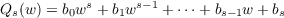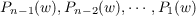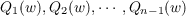#### Vol. 30, No. 2, 1969

 Download this articleFor screen For printingRecent Issues Vol. 325: 1 Vol. 324: 1  2 Vol. 323: 1  2 Vol. 322: 1  2 Vol. 321: 1  2 Vol. 320: 1  2 Vol. 319: 1  2 Vol. 318: 1  2Online Archive Volume: Issue:The Journal Subscriptions Editorial Board Officers Contacts Submission Guidelines Submission Form Policies for Authors ISSN: 1945-5844 (e-only) ISSN: 0030-8730 (print) Special Issues Author Index To Appear Other MSP Journals
An explicit formula for the units of an algebraic number field of degree n 2

### Leon Bernstein and Helmut Hasse

Vol. 30 (1969), No. 2, 293–365
##### Abstract

An infinite set of algebraic number fields is constructed; they are generated by a real algebraic irrational w, which is the root of an equation f(w) = 0 with integer rational coefficients of degree n 2. In such fields polynomials Ps(w) = a0ws + a1ws1 + + as1w + as and( s = 1,,n 1;ak,bk rational integers) are selected so that the Jacobi-Perron algorithm of the n 1 numberscarried out in this decreasing order of the polynomials, and of the n 1 numberscarried out in this increasing order of the polynomials both become periodic.

It is further shown that n 1 different Modified Algorithms of Jacobi-Perron, each carried out with n 1 polynomials Pn1(w),Pn2(w),,P1(w) yield periodicity. From each of these algorithms a unit of the field K(w) is obtained by means of a formula proved by the authors is a previous paper.

It is proved that the equation f(x) = 0 has n real roots when certain restrictions are put on its coefficients and that, under further restrictions, the polynomial f(x) is irreducible in the field of rational numbers. In the field K(w)n 1 different units are constructed in a most simple form as polynomials in w; it is proved in the Appendix that they are independent; the authors conjecture that these n 1 independent units are basic units in K(w).

Primary: 10.65
##### Milestones